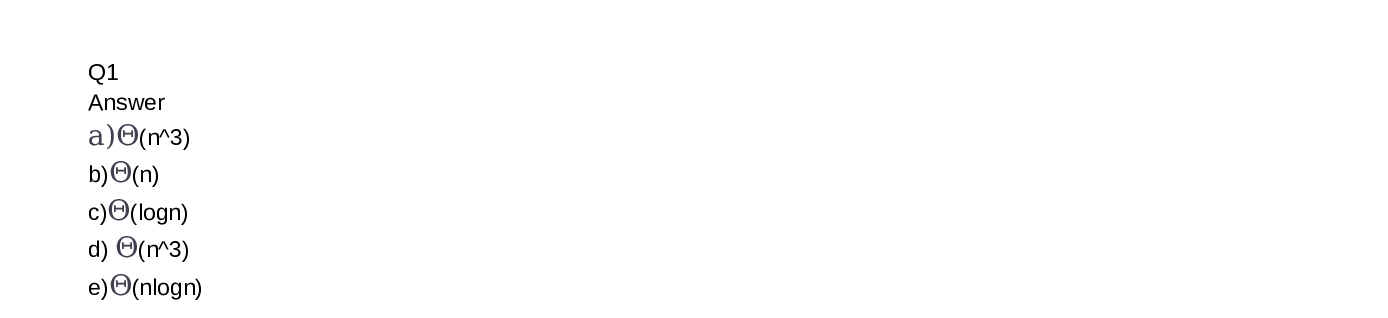Question

Data Structures And Algo

(a) Give the standard Theta (O(. )) form for each of the following functions. (E.g., 2n + 3 would be written as O(n).) [10 marks]

\text { 1. } 5 n^{3}+n^{4}+3 n(\log n)^{10}

\text { 2. } 25 \log n+2 n+7777

\text { 3. } 3 \log \left(n^{4}\right)

\text { 5. } 2 n+3 n(\log n)^{2}+2

\text { 4. } \frac{n(n+1)(2 n+1)}{6}

(b) Put the following Theta classes in order of increasing growth (e.g.,increasing running times). [10 marks]

:) Following are some big-Oh relationships. For each, give witnesses no and c that can be used to prove the relationship. Choose your witnesses to be minimal, in the sense that no – 1 and c are not witnesses, and ifd < c, then no and d are not witnesses. [9 marks]

16 n^{2} \text { is } \mathcal{O}\left(n^{4}\right)

3^{n+5} \text { is } \mathcal{O}\left(3^{2 n}\right)

n^{2} \text { is } \mathcal{O}(n !)Verified### Question 45507Data Structures And Algo

OFor each of the following problems, provide a proof demonstrating the implication.
\mathrm{p} \rightarrow(\mathrm{q} \rightarrow \mathrm{r}) \text { and } \mathrm{p} \rightarrow \mathrm{q} \text { together imply } \mathrm{p} \rightarrow \mathrm{r}
\mathrm{p} \rightarrow(\mathrm{q} \& \sim \mathrm{r}) \text { and }(\mathrm{p} \& \mathrm{q}) \rightarrow \mathrm{r} \text { together imply } \sim \mathrm{p}
\mathrm{q} \rightarrow \mathrm{r} \text { and } \mathrm{p} \mathrm{v} \text { q together imply }(\mathrm{p} \rightarrow \mathrm{q}) \rightarrow(\mathrm{r} \& \mathrm{q})
p \rightarrow(q \vee r) \text { implies }(p \rightarrow q) \vee(p \rightarrow r)

### Question 45506Data Structures And Algo

2) For each of the following pairs of sentences, prove that the first implies the second without using the DM rule - that is, no line in your proof should cite DM.
а)p v (q&r)(p v q) & (p v r)
а2)(p v q) & (p v r)pv (q&r)
p&q(-p v ~q)b)
c)p v q(-p&~q)
d)~p v q
e)-(p→q)p&-q (EXTRA CREDIT)
f)pvq

### Question 44769Data Structures And Algo

Determine the running times of the following function (as tight upperbounds). Justify your answers. You may give the answer in O(...) form. In addition, describe in words what this function computes.3.
Function fnA, given below, if both arrays arrayA and arrayB contain n elements and both arrays are already sorted. The function binary Search execute s the usual binary search algorithm.

### Question 44768Data Structures And Algo

(a) Insert the following items into a BST, in the following order: [18, 14,17, 42, 11, 25, 15, 47). Show the resulting tree. [6 marks]
(b) Put the following items into the BST in Figure la [20, 25, 26, 30, 35,40, 42, 47, 60]. Is this always unique for a fixed array and a fixed BST?Justify your answer. [6 marks]
(c) Mark all non-balanced nodes in the BST in Figure la. Justify your answer. 6 marks]
(d) In the BST you got in the part (b) delete the following items, in the following order: [40, 35, 25] (So when deleting 35, 40 is not in the tree any-more ...). Show how the BST changes in each deletion. [6 marks]
(e) Consider the BST in Figure lb. The node 25 can be raised to be the root of the BST using 2 rotations ( each of the rotations "promotes"25 to the place of its parent). Show the state of the tree after each of these rotations. [6 marks]

### Question 44767Data Structures And Algo

(a) Give the standard Theta (O(. )) form for each of the following functions. (E.g., 2n + 3 would be written as O(n).) [10 marks]
\text { 1. } 5 n^{3}+n^{4}+3 n(\log n)^{10}
\text { 2. } 25 \log n+2 n+7777
\text { 3. } 3 \log \left(n^{4}\right)
\text { 5. } 2 n+3 n(\log n)^{2}+2
\text { 4. } \frac{n(n+1)(2 n+1)}{6}
(b) Put the following Theta classes in order of increasing growth (e.g.,increasing running times). [10 marks]
:) Following are some big-Oh relationships. For each, give witnesses no and c that can be used to prove the relationship. Choose your witnesses to be minimal, in the sense that no – 1 and c are not witnesses, and ifd < c, then no and d are not witnesses. [9 marks]
16 n^{2} \text { is } \mathcal{O}\left(n^{4}\right)
3^{n+5} \text { is } \mathcal{O}\left(3^{2 n}\right)
n^{2} \text { is } \mathcal{O}(n !)

### Question 35357Data Structures And Algo

______is the process of determining the degree to which a software product fulfills its specifications.
D. Program Verification
B. Program Testing
A. Compile errors
C. Design errors

### Question 35356Data Structures And Algo

A step-by-step description of the solution to a problem, is called:
О В. Array
O C. Flowchart
A. Data Type
D. Algorithm

### Question 35355Data Structures And Algo

In ADT Transformer responsible of :
Select one:
D. Observe state
C. Create List
B. Process all components
A. Change state

### Question 35354Data Structures And Algo

________-It assumes that the lower-levels work correctly.
B. Çompiler errors
C. Top down testing approach
D. Integration
A. Bottom up testing approach

### Question 35353Data Structures And Algo

Based on the below code the missing part is:bool Unsorted Type ::

### Submit query